In :
%pylab inline

Populating the interactive namespace from numpy and matplotlib

In :
from __future__ import division
import numpy as np
from IPython.core.display import HTML
from deltasigma import *


# Audio demo of mod1 and mod2 - demo #4¶

Demonstration of the first and second order modulators, as in dsdemo4.m in the MATLAB Delta Sigma Toolbox, albeit this demo employs its Python port deltasigma.

Port written by G. Venturini. First version Jan 1st, 2014. Happy new year!!

## Introduction¶

• The user selects:
• A modulator. It may be the simple mod1 or mod2, or a user specified modulator.
• The source (sine, ramp or file).
• The $\mathrm{sinc}^n$ filter order $n$ and decimation factor.
• The output signal and quantization error are evaluated.
• The signals are passed through the decimation filter.

Input, output and quantization error are compared in terms of time evolution, spectra and played out aloud.

#### Options and utility functions¶

In :
plotsize = (20, 4) #The plot size in inches. Reduce for low res/small screens

In :
# An in-browser HTML5 audio player
# Notice there is an audio player in the dev branch of iPython,
# it is unlikely that as of today Jan 1st, 2014 many people have access to it
# for that reason, the following function is used instead.
# It is originally from the notebook 'the sound of Hydrogen' by 'filmor', with small modifications.
# http://nbviewer.ipython.org/url/gist.github.com/filmor/c7ae1a867fc9058ffcd4/raw/91ce69c1400540ed39f68bd92234abfb1dc2ae70/tone-generator.ipynb
from io import BytesIO
import base64, struct

def wavPlayer(data, rate, scale=False, autoplay=False):
"""This method will display html 5 player for compatible browser with
embedded base64-encoded WAV audio data.

Parameters :
------------
data : 1d np.ndarray containing the audio data to be played
rate : the data rate in Hz
scale : if set to True, the audio signal is amplified to cover the full scale.
"""
if np.max(abs(data)) > 1 or scale:
data = data/np.max(abs(data))
data = (2**13*data).astype(np.int16)

buffer = BytesIO()
buffer.write(b'RIFF')
buffer.write(b'\x00\x00\x00\x00')
buffer.write(b'WAVE')

buffer.write(b'fmt ')
if data.ndim == 1:
noc = 1
else:
noc = data.shape

bits = data.dtype.itemsize * 8
sbytes = rate*(bits // 8)*noc
ba = noc * (bits // 8)
buffer.write(struct.pack('<ihHIIHH', 16, 1, noc, rate, sbytes, ba, bits))

# data chunk
buffer.write(b'data')
buffer.write(struct.pack('<i', data.nbytes))

if data.dtype.byteorder == '>' or (data.dtype.byteorder == '=' and sys.byteorder == 'big'):
data = data.byteswap()

buffer.write(data.astype(np.int16).tostring())

# Determine file size and place it in correct position at start of the file.
size = buffer.tell()
buffer.seek(4)
buffer.write(struct.pack('<i', size-8))

val = buffer.getvalue()
autoplay = " autoplay=\"autoplay\""*autoplay + ""

src = """<audio controls="controls" style="width:600px"{autoplay}>
<source controls src="data:audio/wav;base64,{base64}" type="audio/wav" />
Your browser does not support the audio element.
</audio>""".format(base64=base64.b64encode(val).decode("ascii"), autoplay=autoplay)
display(HTML(src))


## Parameters¶

### Source type¶

Set the SourceType flag, which has the following meaning:

• 0: sine wave, see below for amplitude and frequency values.
• 1: ramp from -.7 to +.7.
• 2: audio file: a 9 seconds long recording of a tenor sax playing. Please make sure you download and save the file sax.wav.b64 first, as explained below.
In :
SourceType = 0 # change the SourceType


If you set SourceType = 0 above, here you can specify the amplitude and frequency of the sine wave.

Otherwise, the following values are ignored.

In :
SineAmp = .4
SineFreq = .5e3


If you set SourceType = 1 (ramp), there is nothing you need to do.

if you set SourceType = 2 (file), make sure you download the file sax.wav.b64 included with this notebook and save it to the current working directory.

### Modulator order¶

Select a modulator order equal to 1 to have the mod1 structure, modulator order equal to 2 provides mod2.

Note: two convenience functions mod1() and mod2() are available to quickly access NTF, STF and ABCD matrix for mod1 and mod2.

Modify the following code to employ your modulator of choice!

In :
mod_order = 2
if not mod_order in (1, 2):
raise ValueError('Please choose an order equal to either 1 or 2')
ABCD_mod1 = np.array([[1., 1., -1.], [1., 0., 0.]])
ABCD_mod2 = np.array([[1., 0., 1., -1.], [1., 1., 1., -2.], [0., 1., 0., 0.]])
ABCD = ABCD_mod1 if mod_order == 1 else ABCD_mod2
print "Delta sigma modulator ABCD matrix:"
print ABCD

Delta sigma modulator ABCD matrix:
[[ 1.  0.  1. -1.]
[ 1.  1.  1. -2.]
[ 0.  1.  0.  0.]]


### $\mathrm{sinc}^n$ decimation filter: parameters¶

In :
SincOrder = 2 # SincOrder
DecFact = 32 # 32 DecFact


### Misc. options¶

In :
T = 2 # Input signal duration in seconds.
FsOut = 8192 # set to ensure compatibility.
Fs = FsOut*DecFact #Fs


## Simulation¶

### Setup the input signal¶

In :
N = int(np.round(T*Fs))
if SourceType == 0:
SineAmp = max(min(SineAmp, 1), 0) # 0 <= SineAmp <= 1
if SineFreq >= FsOut/2:
raise ValueError('Anything above FsOut/2 (%g) will be inaudible.' % FsOut/2)
print "Generating a sine wave:"
print " * at freq. %g Hz, " % SineFreq
print " * with norm. amplitude %g," % SineAmp
print " * sampled at %g Hz," % Fs
print " * total length %g s (%d #samples)." % (T, N)
u = SineAmp*np.sin(2*np.pi*SineFreq/Fs*np.arange(N))*ds_hann(N)
u0 = u[::DecFact]
elif SourceType == 1:
u = np.linspace(-0.7, 0.7, N)
u0 = u[::DecFact]
print "Generating a ramp:"
print " * from -.7 to +.7, "
print " * sampled at %g Hz," % Fs
print " * total length %g s (%d #samples)." % (T, N)
elif SourceType == 2:
from scipy.interpolate import interp1d as interp
filename = 'sax.wav'
T = 9
try:
from scipy.io import wavfile
except ImportError:
print "Error: Reading audio files requires scipy > v 0.12.0"
try:
fpin = open(filename + '.b64', 'r')
fpin.close()
fpout = open(filename, 'wb')
fpout.write(base64.b64decode(b64data))
fpout.close()
except IOError:
if len(filedata.shape) == 2:
filedata = np.average(filedata, axis=1)
T = min(T, filedata.shape/sr)
N = int(np.round(T*Fs))
filedata = filedata[:int(np.round(T*sr)+1)]
if max(abs(filedata)) > 1:
filedata = filedata/max(abs(filedata))
filets = np.arange(filedata.shape)/sr
ts = (np.arange(N)/Fs)
u0 = interp(filets, filedata)(ts[::DecFact])
u = interp(filets, filedata)(ts)
print "Decoded the file %s.b64 and loaded its data." % filename
print "Input data available:"
print " * sampled at %g Hz," % Fs
print " * total length %g s (%d #samples)." % (T, N)
print " * normalized (only if amp > 1)"

Generating a sine wave:
* at freq. 500 Hz,
* with norm. amplitude 0.4,
* sampled at 262144 Hz,
* total length 2 s (524288 #samples).


## Inspect the input signal¶

### Plot: time domain¶

In :
plot(np.arange(N)[::DecFact]/Fs, u0)
figureMagic(size=(20,4))
ylabel('$u(t)$');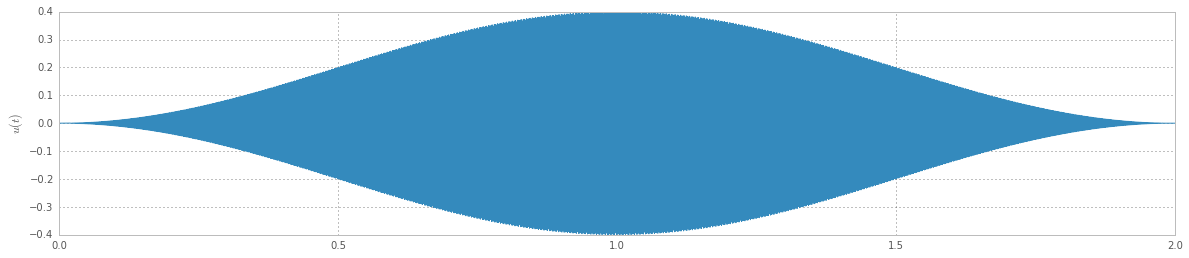### Plot: frequency domain¶

In :
if SourceType == 0 or SourceType == 2:
N = max(u0.shape)
if SourceType == 0:
U = np.fft.fft(u0)/(N/4)
else:
U = np.fft.fft(u0 * ds_hann(N))/(N/4)
f = np.linspace(0, FsOut, N + 1)
f = f[:N/2 + 1]
semilogx(f, dbv(U[:N/2 + 1]))
xlabel('f [Hz]')
ylabel('U(f) [dB]')
figureMagic(xRange=[1, max(f)], size=plotsize, name='Spectrum')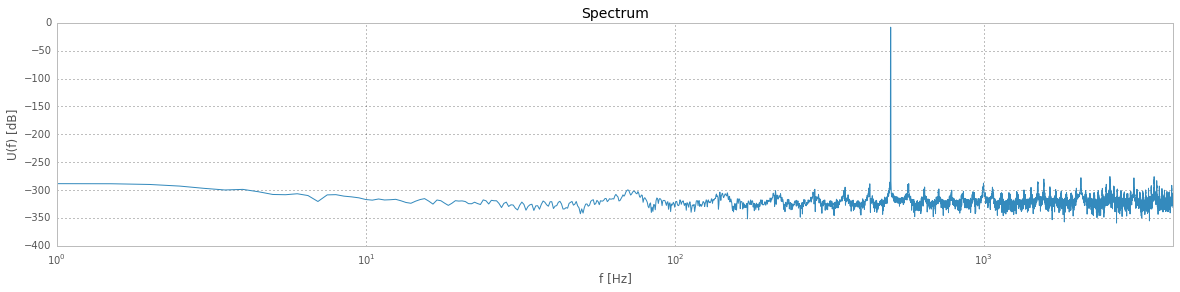### Play the input signal¶

Click on the Play button to listen to the input signal.

Requires a HTML5-capable browser such as recent version of Chrome, Firefox or IE.

In :
# Show a Html 5 audio player
wavPlayer(data=u0, rate=FsOut)


## Simulate!¶

The simulation is run calling simulateDSM().

In :
v, junk1, junk2, y = simulateDSM(u, ABCD)
del junk1, junk2
q = v - y # quantization error


## Inspect the output¶

### Plot: time domain¶

In :
N = max(v.shape)
nPlot = 400
if N > nPlot:
n = np.arange(int(np.floor(N/2 - nPlot/2)), int(np.floor(N/2 + nPlot/2)))
else:
n = np.arange(N)
n = n.astype(np.int32)
hold(True)
t = np.arange(max(n.shape))
step(t, u[n], 'r')
bar(t, v[n], color='b', linewidth=0)
ylabel('$u(t), v(t)$')
xlabel('Sample #')
axis([0, max(n)-min(n), -1.1, 1.1])
figureMagic(size=(20, 4), name='Modulator Input & Output')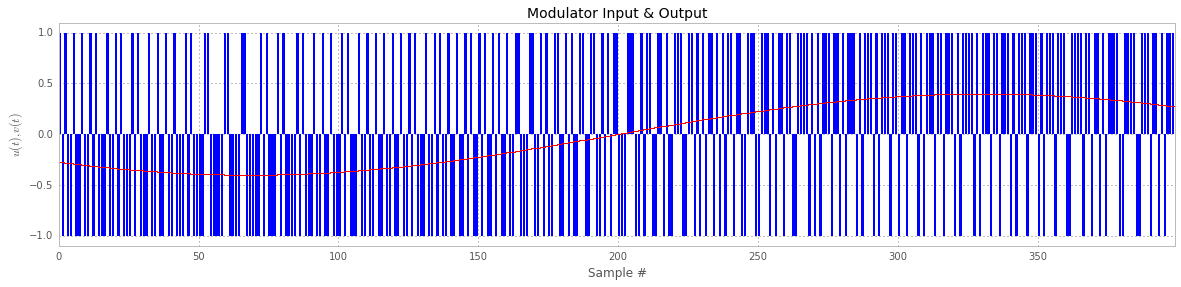### Plot: frequency domain¶

In :
N = max(v.shape)
Nfft = min(N, 16*8192)
n = np.arange((N - Nfft)/2, (N + Nfft)/2).astype(np.int32)
V = np.fft.fft(v[n] * ds_hann(Nfft)) / (Nfft / 4)
if SourceType == 1:
inBin = np.round(SineFreq/Fs*Nfft)
else:
inBin = np.ceil(Nfft/1000)
hold(True)
ylabel('V(f) [dB]')
xlabel('Frequency [Hz]')
semilogx(np.arange(max(V.shape))/max(V.shape)*Fs, dbv(V))
f, Vp = logsmooth(V, inBin)
semilogx(f*Fs, Vp, 'm', linewidth=2.5)
xlim([f*Fs, Fs/2])
msg = 'NBW = %.1f Hz ' % (Fs*1.5/Nfft)
text(Fs/2, -90, msg, horizontalalignment='right', verticalalignment='center')
figureMagic(size=plotsize, name='Spectrum')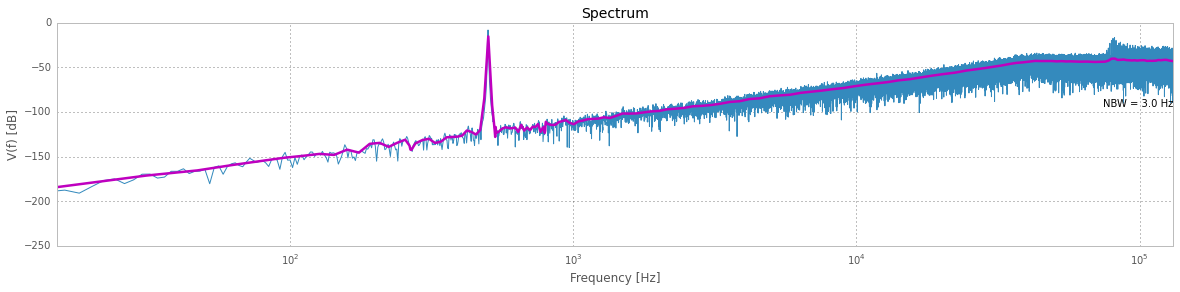### Decimate; Comparison of output signal and input signal¶

In :
w = sinc_decimate(v, SincOrder, DecFact)
filtered_q = sinc_decimate(q, SincOrder, DecFact)
N = max(w.shape)
t = np.arange(N)/FsOut
subplot(211)
plot(t, w)
ylabel('$w$')
figureMagic(size=(20, 4))
subplot(212)
plot(t, u0 - w, 'g')
ylabel('$u-w$')
xlabel('t [s]')
figureMagic(size=(20, 4))
suptitle('Output and conversion error');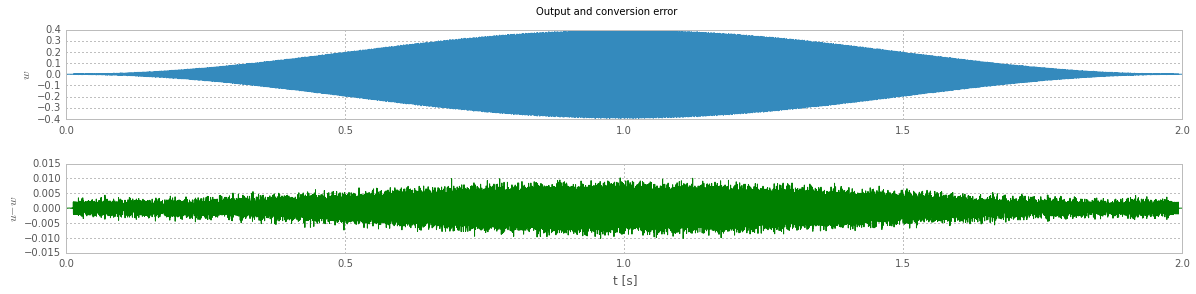### Play the output signal¶

Click on the Play button to listen to the output signal w.

In :
wavPlayer(data=w, rate=FsOut)


### Play the quantization error (filtered)¶

Click on the Play button to listen to the quantization error filtered_q.

In :
wavPlayer(data=filtered_q, rate=FsOut, scale=True)


### Play the difference in the signal before and after A/D conversion¶

In :
wavPlayer(data=u0-w, rate=FsOut, scale=True)


### Final comparison of the spectra of the three signals¶

In :
N = max(filtered_q.shape)
Nfft = min(N, 16*8192)
n = np.arange((N - Nfft)/2, (N + Nfft)/2).astype(np.int32)
E = np.fft.fft(filtered_q[n] * ds_hann(Nfft)) / (Nfft / 4)
W = np.fft.fft(w[n] * ds_hann(Nfft)) / (Nfft / 4)
U0 = np.fft.fft(u0[n] * ds_hann(Nfft)) / (Nfft / 4)
if SourceType == 0:
inBin = np.round(SineFreq*Nfft)/FsOut
else:
inBin = np.ceil(Nfft/1000)
hold(True)
ylabel('dB')
semilogx(np.arange(Nfft)/Nfft*FsOut, dbv(U0), label='Input signal')
semilogx(np.arange(Nfft)/Nfft*FsOut, dbv(W), label='Output signal')
semilogx(np.arange(Nfft)/Nfft*FsOut, dbv(E), label='Filtered quant. error')
f, U0p = logsmooth(U0, inBin)
semilogx(f*FsOut, U0p, '#1E90FF', linewidth=2.5)
f, Wp = logsmooth(W, inBin)
semilogx(f*FsOut, Wp, '#556B2F', linewidth=2.5)
f, Ep = logsmooth(E, inBin)
semilogx(f*FsOut, Ep, '#8B0000', linewidth=2.5)
xlim([10, FsOut/2])
msg = 'NBW = %.1f Hz ' % (Fs*1.5/Nfft)
text(FsOut/2, -6, msg, horizontalalignment='right', verticalalignment='top')
figureMagic(size=plotsize, name='Spectrum')
legend(loc=3);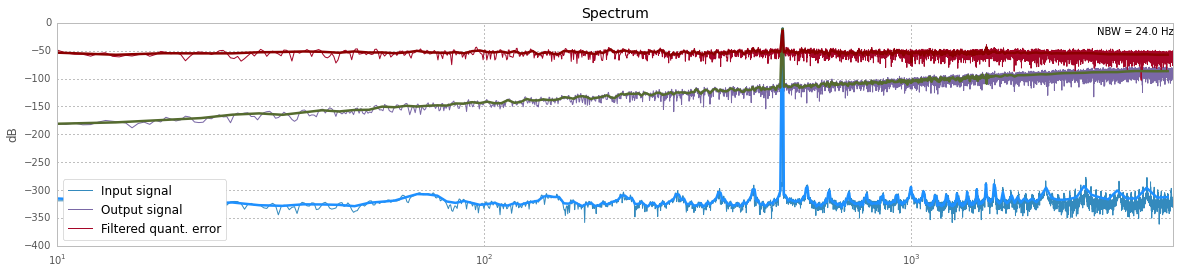## Conclusions¶

We briefly demonstrated the use of two modulators to perform A/D conversion of audio signals.

Feel free to go through this notebook, change the parameters and resimulate!

### System version information¶

In :
#%install_ext http://raw.github.com/jrjohansson/version_information/master/version_information.py

%version_information numpy, scipy, matplotlib, deltasigma

Out:
SoftwareVersion
Python2.7.10 64bit [GCC 4.2.1 (Apple Inc. build 5577)]
IPython3.2.1
OSDarwin 14.4.0 x86_64 i386 64bit
numpy1.9.2
scipy0.16.0
matplotlib1.4.3
deltasigma0.2.2
Fri Aug 07 16:21:14 2015 CEST
In [ ]: